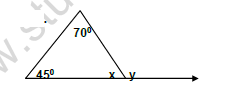# CBSE Class 7 Mathematics Worksheet Set K Solved

Download printable Mathematics Class 7 Worksheets in pdf format, CBSE Class 7 Mathematics Worksheet Set K Solved has been prepared as per the latest syllabus and exam pattern issued by CBSE, NCERT and KVS. Also download free pdf Mathematics Class 7 Assignments and practice them daily to get better marks in tests and exams for Grade 7. Free chapter wise worksheets with answers have been designed by Standard 7 teachers as per latest examination pattern

## Mathematics Worksheet for Class 7

Class 7 Mathematics students should refer to the following printable worksheet in Pdf in Grade 7. This test paper with questions and solutions for Standard 7 Mathematics will be very useful for tests and exams and help you to score better marks

### Class 7 Mathematics Worksheet Pdf

SECTION A

Q.NO.1 FILL IN THE BLANKS

(a) Two angles of a triangle are 60 0 and 25 0 ,the measure of third angle is ______.

(b) The longest side in the ΔPQR ,right angled at Q is ___________________.

(c) The exterior angle of a triangle is 105 0 , the measure of its adjacent interior angle is _______________

SECTION B

Q.NO.2

(a) Construct ΔPQR in which PQ = 6cm , QR = 3.5cm and PR =5cm.

(b) Find the values of x, y . Give reasons.(c) Is it possible to draw a triangle with sides 7.5cm , 3.5cm , 5.5cm. Give reason.

(d) Find the length of the side PQ in the right triangle PQR with sides QR=8cm, PR =10cm and /Q = 90 0.

SECTION - C

Q.NO

3 Construct ΔABC in which BC=5cm , AB= 8cm and /C =90 0

4 A 20m long ladder reached a window 16m high from the ground on placing it against a wall at a certain distance. Find the distance of the foot of the ladder from the wall.

5 Draw a line AB and consider a point P not on it. Through P , draw a line XY parallel to line AB.